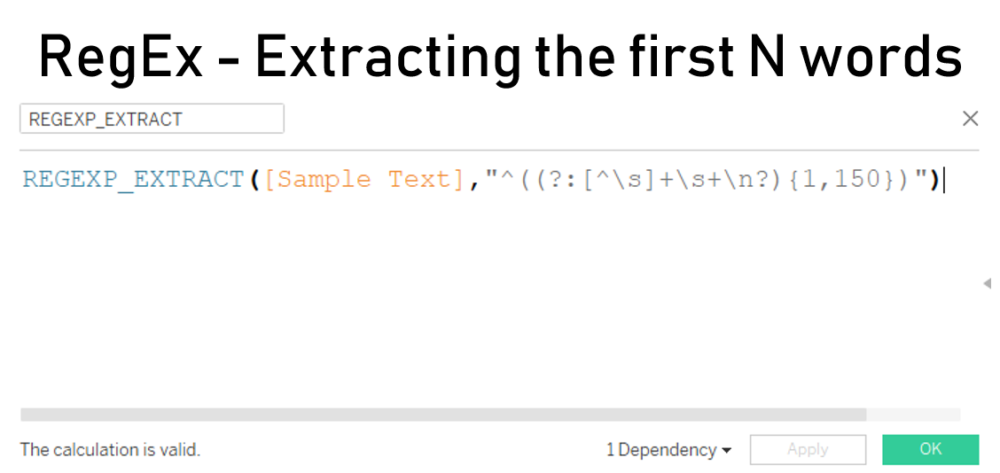## RegEx – Extracting the first N words

I was recently asked by a colleague for some help with a RegEx expression for extracting the first N number of words from a block of text. In this blog, I’ll explain how to create an expression for this task & how to set it up in both Alteryx and Tableau.

## THE EXPRESSION

First we need to create a regular expression to match the first 150 words. Since we want to take the words at the start, we can use a ^ symbol to start as this represents the beginning of a string.

Next we want to identify a set of word characters. These could include letters, numbers or even punctuation in the form of full stops or commas. For this we essentially want to look for any character that is NOT a space. For this we can use \S (backslash uppercase s). We can add a + after so that we match one or more characters.

^\S+

Then to identify the spaces between our words, we want to look for a \s (backslash lowercase s). Again, we will follow this with a + in case the text has more than one space between words.

^\S+\s+

Finally, to let us capture words across paragraphs, we can use a \n to match new-line characters, or carriage returns. This will need to be followed by a ? as not every word will be followed by a new line, so this makes the character optional.

^\S+\s+\n?

Now that we have the basic structure for a single word, we need to specify how many of these to match using curly brackets. In this example, I want to capture from the first word to the 150th word: {1,150}. To make this apply to the entire word group, we will need to use a capture group to assign the {1,150} to the word.

^(\S+\s+\n?){1,150}

However we don’t want to actually capture an individual word, but the entire set of the first 150 words. Therefore, we’ll need to convert that capture group into a non-capturing group using ?: at the start of the group.

^(?:\S+\s+\n?){1,150}

Finally, we can wrap the entire expression in a capture group so that we can match the first 150 words. So now our completed expression looks like this:

(^(?:\S+\s+\n?){1,150})

## TABLEAU

To extract this text in Tableau is not too taxing. We will need to create a new calculated field which will contain our extracted text, extracting the text from an existing field in our data. That field will be created using the REGEXP_EXTRACT function, alongside the expression we created earlier.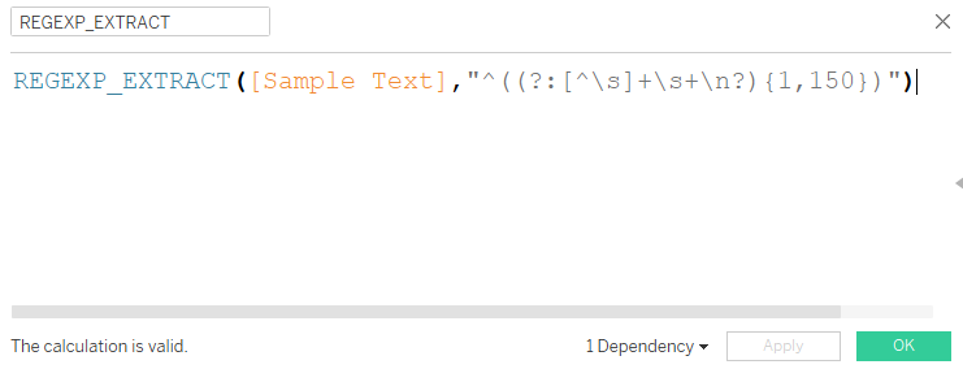This is the calculation used to extract the first 150 words from the Sample Text field.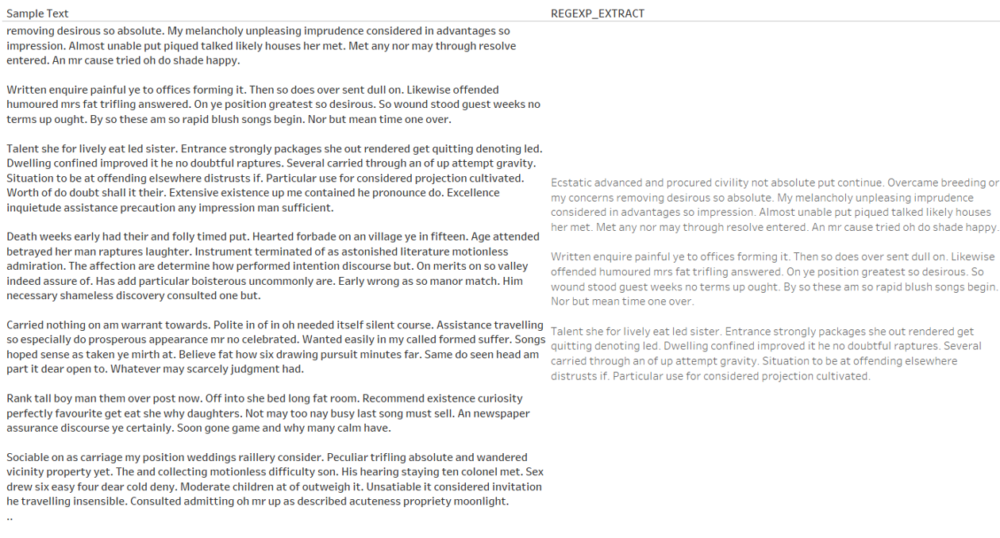This is a comparison between the Sample Text field and the field containing the first 150 words, created using the above calculated field.

## ALTERYX

Using this in Alteryx is also quite straightforward. All we need to do is use a RegEx tool in parse mode.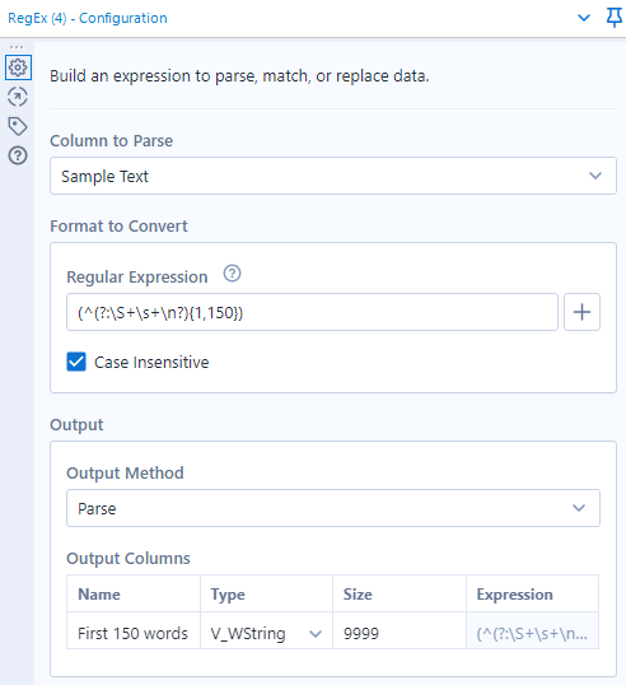Here is the configuration for the RegEx tool in Alteryx to extract the first 150 words from our sample text.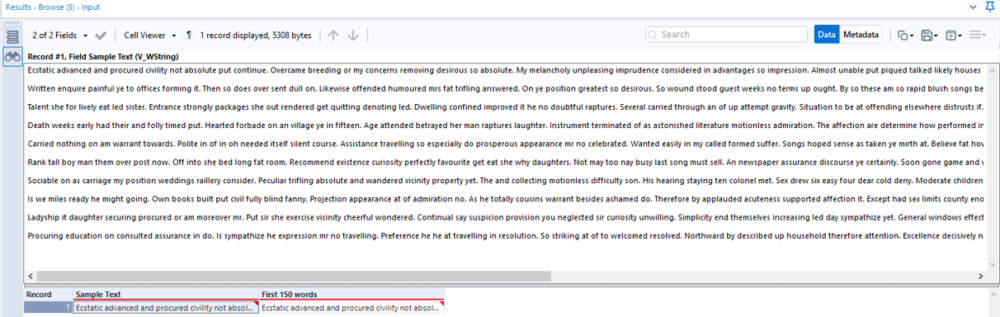This is our sample text field in Alteryx. Here the lines are too long to display all of them together as the cell preview doesn’t wrap text.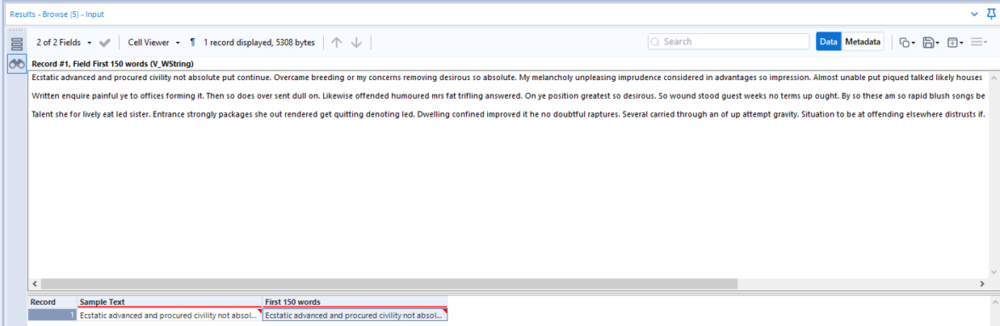Here is the field created by the RegEx tool, extracting the first 150 words from our sample text.

And there you have it! Even if you don’t have need for the use case, I hope the logic of creating the expression is useful to help improve your understanding of how RegEx works.

London, UK